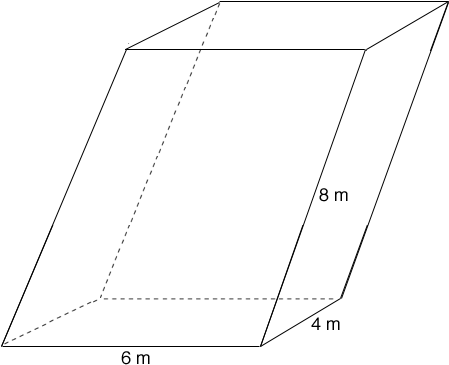SEARCH HOMEMath Central Quandaries & QueriesQuestion from ryan, a student: Hey guys! Got a question, I'm really confused what the question mean and how the diagram would look like... someone please help... Here's the question. The base of a parallelepiped is a rectangle 4m by 6m. If its lateral edge is 8m and is inclined at an angle 45 degrees to a 6m edge of the base, find the total area and volume of its parallelepiped. My dilemma is what does it mean by the lateral edge of 8m is inclined at 45 degrees to a 6m edge of the base? Can someone post a diagram to help me solve the problem. Thanks in advance. ryan7Ryan,

There are two things wrong with the statement of the problem, so it is not surprising that you are confused. So am I! The word "lateral" is not the right word -- it means "on the side" or "to the side", which is meaningless here. We can probably guess what is intended by the question, but first we should make sure we know what a parallelepiped is: it is a polyhedron with 6 faces (like a cube), but the opposite pairs of faces are congruent parallelograms. In your problem we are told that the bottom of your parallelepiped is a 4 by 6 rectangle, and therefore so is the top. We are told that another pair of faces, say front and back, are parallelograms with sides of length 8 and 6 that come together at a 45 degree angle. Unfortunately we are not told the shape of the remaining pair of faces (left and right). We must GUESS that it was intended for the front and back faces to be vertical, so that the remaining faces are 4 by 8 rectangles (slanted at a 45 degree angle to the base plane).We can now find the volume, which is equal to the area of the base times the height:

AREA (base) = 4 X 6 = 24 square m.

I'll let you find the height -- which is the length of a leg of an isosceles right triangle whose hypotenuse is 8.

The volume is then 24*h cubic m. But remember we had to guess that the front and back faces are in vertical planes. Otherwise, there is insufficient information to determine the volume!

ChrisMath Central is supported by the University of Regina and The Pacific Institute for the Mathematical Sciences.